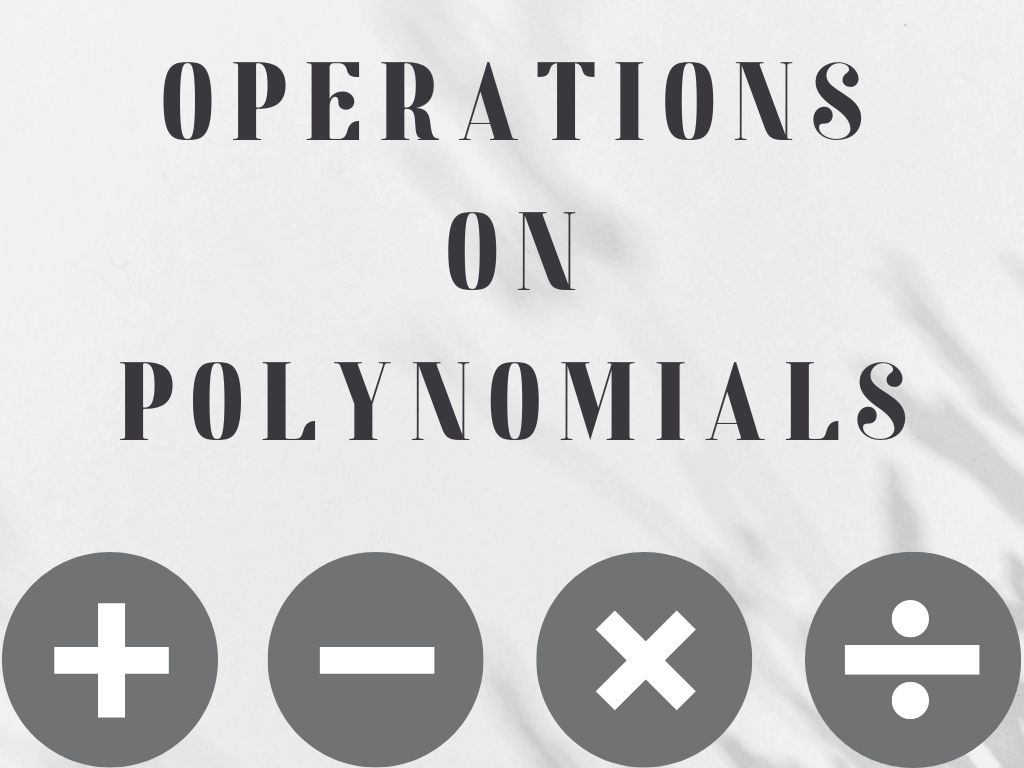## Operations on Polynomials Class 10th

Introduction Operations on polynomials are the addition of polynomials, the subtraction of polynomials, the multiplication of polynomials, and the division of polynomials. ➤ Addition of polynomials ➤ Subtraction of polynomials ➤ Multiplication of polynomials ➤ Division of polynomials Addition of Polynomials When we add two or more polynomials then only like terms are added. it …